Chapter 13 Class 10 Surface Areas and Volumes

Class 10
Important Questions for Exam - Class 10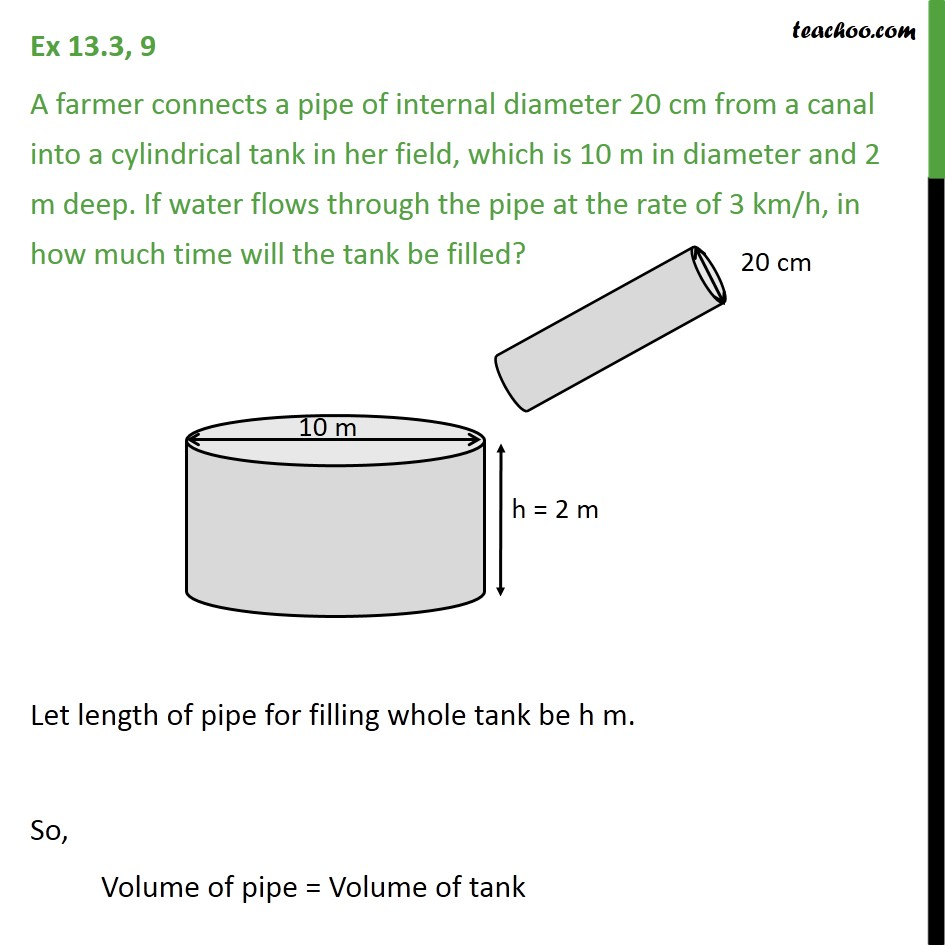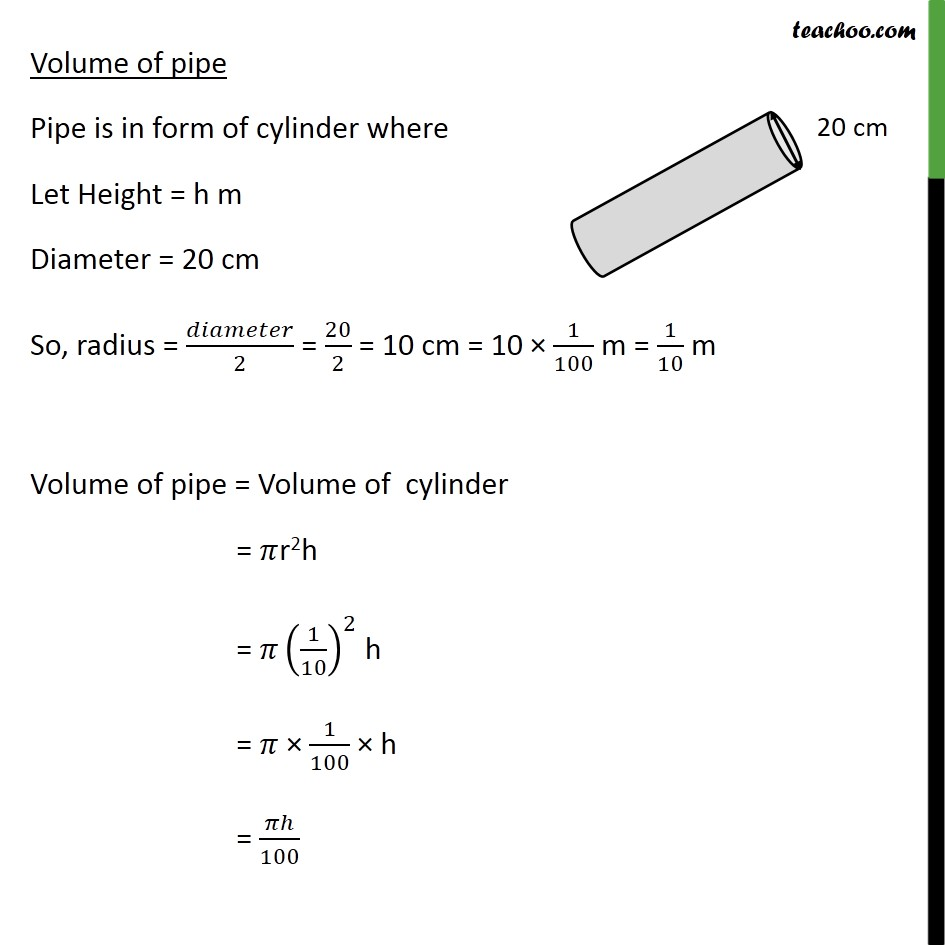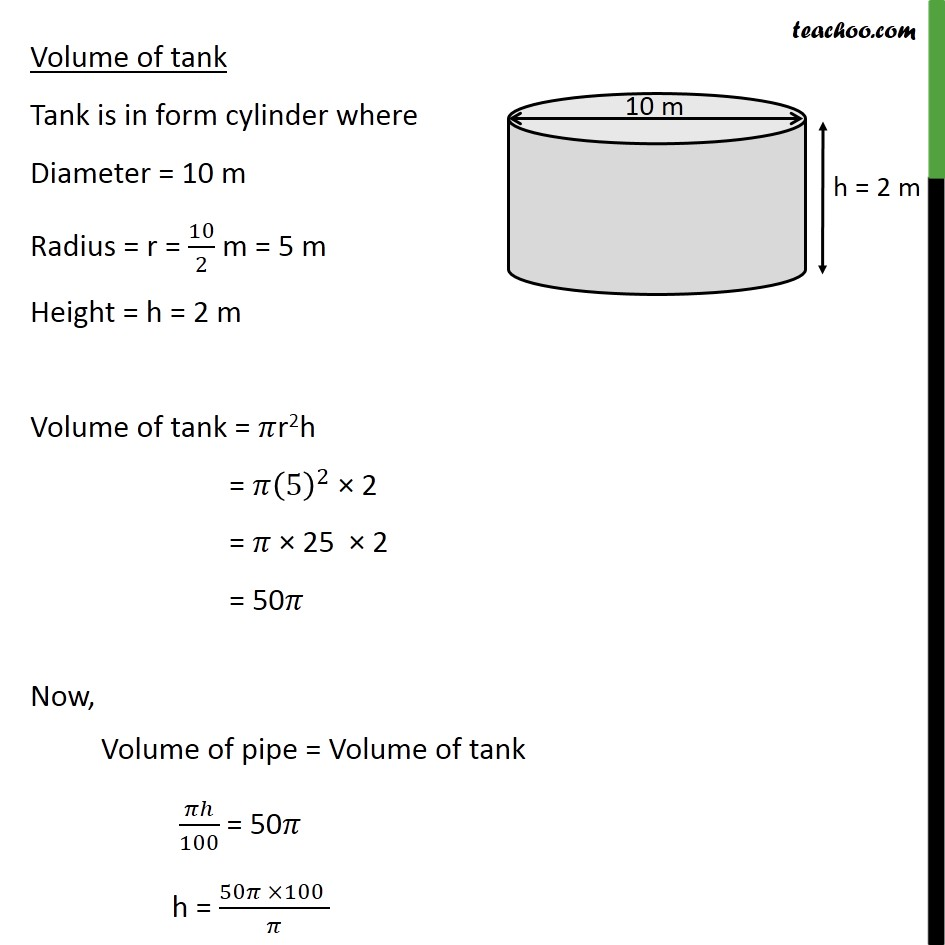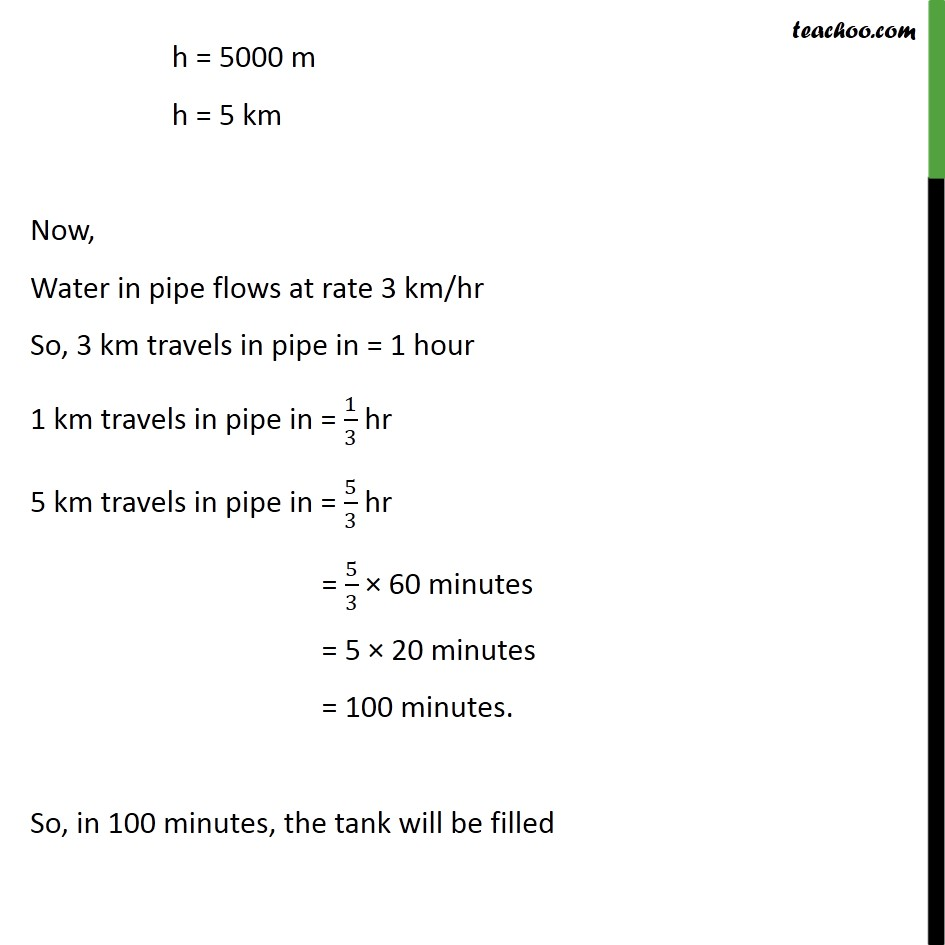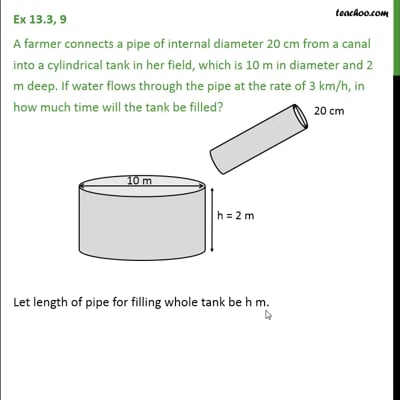This video is only available for Teachoo black users

Solve all your doubts with Teachoo Black (new monthly pack available now!)

### Transcript

Ex 13.3, 9 A farmer connects a pipe of internal diameter 20 cm from a canal into a cylindrical tank in her field, which is 10 m in diameter and 2 m deep. If water flows through the pipe at the rate of 3 km/h, in how much time will the tank be filled? Let length of pipe for filling whole tank be h m. So, Volume of pipe = Volume of tank Volume of pipe Pipe is in form of cylinder where Let Height = h m Diameter = 20 cm So, radius = 𝑑𝑖𝑎𝑚𝑒𝑡𝑒𝑟/2 = 20/2 = 10 cm = 10 × 1/100 m = 1/10 m Volume of pipe = Volume of cylinder = 𝜋r2h = 𝜋(1/10)^2 h = 𝜋 × 1/100 × h = 𝜋ℎ/100 Volume of tank Tank is in form cylinder where Diameter = 10 m Radius = r = 10/2 m = 5 m Height = h = 2 m Volume of tank = 𝜋r2h = 𝜋(5)^2 × 2 = 𝜋 × 25 × 2 = 50𝜋 Now, Volume of pipe = Volume of tank 𝜋ℎ/100 = 50𝜋 h = (50𝜋 ×100 )/𝜋 h = 5000 m h = 5 km Now, Water in pipe flows at rate 3 km/hr So, 3 km travels in pipe in = 1 hour 1 km travels in pipe in = 1/3 hr 5 km travels in pipe in = 5/3 hr = 5/3 × 60 minutes = 5 × 20 minutes = 100 minutes. So, in 100 minutes, the tank will be filled